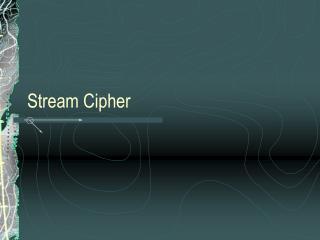DownloadDownload PresentationStream Cipher

# Stream Cipher

Download Presentation## Stream Cipher

- - - - - - - - - - - - - - - - - - - - - - - - - - - E N D - - - - - - - - - - - - - - - - - - - - - - - - - - -
##### Presentation Transcript

1. Stream Cipher

2. Stream Cipher • A stream cipher breaks the message M into successive characters or bits m1, m2, ..., and enciphers each mi with the ith element ki of a key stream K=k1k2...; that is, • EK(M)=Ek1(m1)Ek2(m2)...

3. Periodic • A stream cipher is periodic if the key stream repeats after d characters for some fixed d; otherwise, it is nonperiodic. • Periodic: • Rotor cipher, Hagelin cipher • Nonperiodic: • Vernam cipher (one-time pad), running-key cipher

4. Stream Cipher • Two different approaches： • synchronous methods • self-synchronous methods

5. Synchronous Stream Cipher • The key stream is generated independently of the message stream. • If a ciphertext character is lost during transmission, the sender and receiver must resynchronize their key generators before they can proceed further.

6. Synchronous Stream Cipher • Must ensure no part of the key stream is repeated • Linear Feedback Shift Registers • Output-block Feedback Mode • Counter Method

7. Example of SSC

8. Self-synchronous Methods • Each key character is derived from a fixed number n of preceding ciphertext characters. • If a ciphertext character is lost or altered during transmission, the error propagates forward for n characters, but the cipher resynchronizes by itself after n correct ciphertext character have been received. • Autokey cipher and Cipher Feedback Mode (CFM) • Nonperiodic.

9. Example of Self-synchronous

10. Error Handling • If errors are propagated by the decryption algorithm, applying error detecting codes before encryption provides a mechanism for authenticity.

11. Synchronous Stream Cipher • key stream is generated independently of the message stream • key stream must deterministic so the stream can be reproduced for decipherment. • How to generate a random key stream? • The starting stage of the key generator is initialized by a “seed” I0.

12. Stream Cipher • Stream ciphers are often breakable if the key stream repeats or has redundancy. • To be un breakable, it must be a random sequence as long as the plaintext. • Each element in the key alphabet should be uniformly distributed over the key stream, and there should be no long repeated subsequences or other patterns. • No finite algorithm can generate truly random sequences.

13. LFSR • LFSR (Linear Feedback Shift Register) • shift register R=(rn, rn-1, ..., r1) • “tap” sequence T=(tn, tn-1, ..., t1) • ti and ri are binary digit • bit r1 is appended to the key stream, • bits rn, ...,r2 are shifted right • a new bit derived from T and R is inserted into the left end of the register.

14. LFSR • Letting R’=(rn’, rn-1’, ... r1’) denote the next state of R, we see that the computation of R’ is thus: • ri’=ri+1 i=1,...,n-1 • rn’=TR=∑ni=1tiri mod 2 • R’=HR mod 2, where H is the nxn matrix. • T(x)=tnxn + tn-1xn-1 + ... + t1x + 1 • 若T(x)為質多項式（primitive polynomial）則可以產生2n-1個sequence.

15. LFSR

16. LFSR

17. Example of LFSR

18. Example of LFSR

19. Example of LFSR

20. Example of LFSR

21. LFSR • The feedback loop attempts to simulate a one-time pad by transforming a short key I0 into a long pseudo-random sequence K. • Unfortunately, the result is a poor approximation of the one-time pad.

22. Example of LFSR

23. Cryptanalysis of LFSR • Known-plaintext attack • 2n pairs of plaintext-ciphertext pairs • M=m1...m2n, C=c1...c2n • mici=mi (mi ki)=ki, i=1,...,2n

24. Cryptanalysis of LFSR

25. Output-Block Feedback Mode • weakness of LFSR is caused by the linearity of R’=HR mod 2 • Nonlinear block ciphers such as the DES seem to be good candidates for this.

26. Output-block Feedback Mode

27. Counter Method • Successive input blocks are generated by a simple counter. • It is possible to generate the ith key character ki without generating the first i-1 key characters by setting the counter to I0 + i –1

28. Counter Method

29. Self-Synchronous Stream Cipher • A Self-synchronous stream cipher derives each key character from a fixed number n of preceding ciphertext characters. • Autokey Cipher and Cipher Feedback

30. Autokey Cipher • An autokey cipher is one in which the key is derived from the message it enciphers. • In Vigenere first cipher, the key is formed by appending the plaintext M= m1m2... to a “priming key” character k1; the ith key character (i>1) is thus given by ki=mi-1.

31. Autokey Cipher • In Vigenere second cipher, the key is formed by appending each character of the ciphertext to the priming key k1; that is, ki=ci-1 (i > 1)

32. Aotukey Cipher • 缺點：it exposes the key in the ciphertext stream • This problem is easily remedied by passing the ciphertext characters through a nonlinear block cipher to derive the key characters. • Cipher Feedback mode (CFM)

33. Cipher Feedback mode (CFM) • The ciphertext characters participate in the feedback loop. • It is sometimes called “changing”, because each ciphertext character is functionally dependent on (chained to) preceding ciphertext characters.

34. Example of CFM

35. 亂數產生器 • LFSR • 線性同餘產生器 • 非線性亂數產生器 • 截切亂數產生器 • 數學計算產生器 • 分解因數法 • 離散對數法 • 二次剩餘法 • 質數法

36. LFSR

37. 線性同餘產生器 • xi=axi-1 + b (mod m) • x0為初值 • a, b, m 為KEY • 條件： • gcd(b,m)=1 • 對於每個能夠整除M之質數p而言，b=a-1必須為p 之整數倍 • IF 4|m then 4|b • 缺點：產生之亂數可預測

38. 非線性亂數產生器

39. 截切亂數產生器

40. 亂數產生器的安全性評估 • 好的亂數產生器具備之特性 • 週期長 • 不可預測性（Unpredictable） • 測試法： • Chi-Square 測試法 • Kolmogorov-Smirnov(KS)測試法

41. Chi-Square 測試法

42. 判斷標準